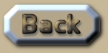Density of the Universe

 Density of the Universe A central reason why it is difficult to determine the density of the Universe is that its shape is quite confusing. Contemporary theories as to the density of the Universe all seem to rest on the starting premise of ‘Critical Density’. Critical Density hinges on the notion that below a certain density, the Universe will contract due to gravity, and above this density, the Universe will expand forever. Sum Theory postulates that the Critical Density of the Universe is irrelevant to whether it will expand or contract. But before analysing the Critical Density, we must look at how the density of how the Universe is either 10^-19 kg/km^3 or 3x10^-18 kg/km^3: .
 To assess this we need to first approximate the Local Galaxy Density: which is the mass of the Milky-way dispersed over a volume of space extending to the nearest major galaxy (Andromeda). 6x10^42kg per 2x10^19km (linear) is distributed over 3d space, which becomes the mass of the Milky-way per 8x10^57km^3. So the average density within our local cluster of galaxies is about 7.5x10^-16kg/km^3. Next we examine the average distribution of galaxies in the Universe, and after perusing numerous accounts of the matter I reach an estimated average that suggests that typically Andromeda is about 6.5 times closer than the average distance between major galaxies. This is because galaxies congregate in clusters. So because we are examining 3d-space, we divide the Local Galaxy Density by 6.5^3 which means that Sum Theory reaches the answer that the Universe has a density of about 2.7x10^-18 kg/km^3. .This is an extract summary of Chapter XXX of the book: Flight Light and Spin